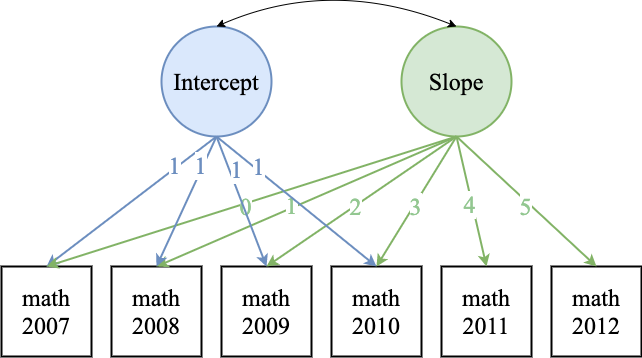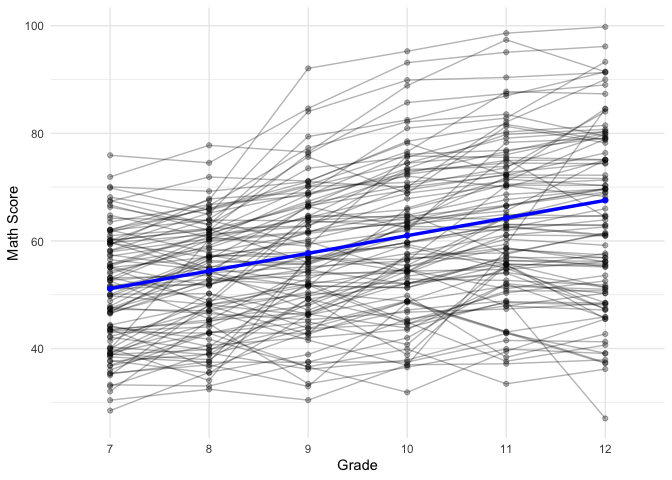# Lab 6 - Latent Growth Models

## Structural Equation Modeling - Instructor: Karen Nylund-Gibson

### May 07, 2020

University of California, Santa Barbara

# Lab preparation

## Creating a version-controlled R-Project with Github

On the Github repository webpage:

1. fork your own branch of the lab repository
2. copy the repository web URL address from the clone or download menu

Within R-Studio:

1. click “NEW PROJECT”
2. choose option Version Control
3. choose option Git
4. paste the repository web URL path copied from the clone or download menu on Github page
5. choose location of the R-Project (too many nested folders will result in filepath error)

## Data sources:

1. The first 3 models utilize a public use data subset the Longitudinal Survey of American Youth (LSAY) $$\color{blue}{\text{See documentation here}}$$

2. The 4th model utilizes a public use data subset the High School Longitudinal Study (HSLS) $$\color{blue}{\text{See documentation here}}$$

library(gganimate)
library(hrbrthemes)
library(tidyverse)
library(haven)
library(janitor)
library(MplusAutomation)
library(rhdf5)
library(here)
library(kableExtra)
library(gtsummary)
library(semPlot)

## LSAY data example - Math Scores across 6 timepoints

lsay_data <- read_spss(here("data", "LSAY_Lab6.sav")) %>% select(-starts_with("AB"),
ends_with("IMP"), -contains("BIO"), -contains("PHY"), -contains("SCI"), FATHED,
MOTHED) %>% clean_names() %>% rename(math_07 = amthimp, math_08 = cmthimp, math_09 = emthimp,
math_10 = gmthimp, math_11 = imthimp, math_12 = kmthimp)

lsay_data[lsay_data == 9999] <- NA

sjPlot::view_df(lsay_data)

Write a CSV file

write_csv(lsay_data, here("data", "lsay_lab6_data.csv"))

Read in the CSV file (SPSS labels removed)

lsay_lab6 <- read_csv(here("data", "lsay_lab6_data.csv"))

Table. LSAY repeated measures
Name Labels
math_07 7th grade math score (imputed)
math_08 8th grade math score (imputed)
math_09 9th grade math score (imputed)
math_10 10th grade math score (imputed)
math_11 11th grade math score (imputed)
math_12 12th grade math score (imputed)## Model 1 - Latent growth model with fixed time effects (equal intervals)

m1_growth  <- mplusObject(
TITLE = "m1 growth model fixed time scores - Lab 6",
VARIABLE =
"usevar =
math_07-math_12; ",

ANALYSIS =
"estimator = ML" ,

MODEL =
"i s | math_07@0 math_08@1 math_09@2 math_10@3 math_11@4 math_12@5; " ,

OUTPUT = "sampstat standardized;",

PLOT = "type=plot3;
series = math_07-math_12(*)",

usevariables = colnames(lsay_lab6),
rdata = lsay_lab6)

m1_growth_fit <- mplusModeler(m1_growth,
dataout=here("mplus_files", "Lab6.dat"),
modelout=here("mplus_files", "m1_growth_Lab6.inp"),
check=TRUE, run = TRUE, hashfilename = FALSE)

Load in the mplus.R functions

source(here("mplus.R.txt"))
##  "Loaded rhdf5 package"

## Plotting using gh5 plot data generated by Mplus

1. View plots available for a given model
2. Generate plots using the get.plot.___ function
3. Extract data and transform to tidy format
4. Plot with ggplot
mplus.view.plots(here("mplus_files", "m1_growth_Lab6.gh5"))

Prepare plot data

observed <- lsay_lab6 %>% select(starts_with("math")) %>%
rownames_to_column() %>% drop_na()

obs100 <- observed[1:100,]

plot_obs <- obs100 %>%
pivot_longer(math_07:math_12, # The columns I'm gathering together
names_to = "grade", # new column name for existing names
values_to = "value") # new column name to store values

mean_est <- as.data.frame(mplus.get.estimated_means(here("mplus_files", "m1_growth_Lab6.gh5"))) %>%
mutate(grade = gradelevels)

Plot the model estimated means superimposted on the obserbed individual values

growth_plot <- ggplot() +
geom_point(data = plot_obs, aes(x = grade, y = value, group = rowname), alpha = .3) +     #
geom_line(data = plot_obs, aes(x = grade, y = value, group = rowname), alpha = .3) +      #
geom_point(data=mean_est, aes(x=grade, y = V1), color = "Blue", size = 1.5) +             #
geom_line(data=mean_est, aes(x=grade, y = V1, group = 1), color = "Blue", size = 1.2) +   #
scale_x_discrete(labels = c("7", "8", "9", "10", "11", "12")) +                           #
growth_plotggsave(here("figures", "spaghetti_p1.png"), height = 6, width = 8, dpi = "retina")
Animate the plot with {gganimate}
growth_plot + transition_states(rowname, transition_length = 1, state_length = 1) +          #
shadow_mark(color = "Magenta", alpha = .3)                                                 #